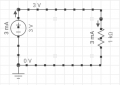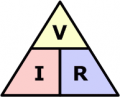# Understanding ohms law

#### Miracletech

Joined Nov 15, 2019
125
In school, we were performing tests where you connect a resistor in parallel with a DC voltage source.
In my case, I am using a 3v DC source and a 2A current source, with a 1k ohm resistance. Is there any way of calculating the voltage and current output from the circuit without a millimeter, and what is this type of circuit called?

#### SamR

Joined Mar 19, 2019
2,259
Simply apply Ohm's law. Current = Voltage/Resistance. You set the V on the power supply, used a resistor marked with it's value R so calculate the I. And it's not in Parallel, it's in series.

#### dl324

Joined Mar 30, 2015
10,985
I am using a 3v DC source and a 2A current source, with a 1k ohm resistance.I don't see a 2A current source.
Is there any way of calculating the voltage and current output from the circuit without a millimeter, and what is this type of circuit called?
I assume "millimeter" means multimeter.

There is no special name for the circuit. You're extremely limited without a meter. Voltage sources and resistors have a tolerance, so you need to take measurements to calculate actual current.

#### Miracletech

Joined Nov 15, 2019
125
But I observed that when I calculated the current,

#### SamR

Joined Mar 19, 2019
2,259
The confusion may be the "2A Source". It may be capable of providing up to 2A but will only provide the current needed by the circuit.

•Rich2

#### Miracletech

Joined Nov 15, 2019
125
It was not same as testing with a multimeter

#### Miracletech

Joined Nov 15, 2019
125
We used a 3v battery (1.5v*2), I measured current from battery using multimeter, it's reading 2.40, I don't know the difference between AH and Amps......

#### bertus

Joined Apr 5, 2008
20,568
Hello,

How did you measure the current?
Current measurements are done in series with the load.
Voltage measurements are done accross the load.

Bertus

#### SamR

Joined Mar 19, 2019
2,259
Amps have to be measured by inserting the multimeter into the circuit. The circuit has to be opened and the meter made part of the circuit. I will need to be on the mA (milliamp) range of the meter. If you used the meter across the battery you are shorting out the battery and may in fact measure 2+A briefly.

#### peterdeco

Joined Oct 8, 2019
185
There is no voltage to calculate. It is 3V. Regardless of how many amps your power supply can provide, the current flowing through the resistor is determined by it's resistance. Your schematic already shows 3mA as the current. This picture is called an Ohm's Law Triangle. It's an easy way to remember V=IxR, I=V/R, R=V/I. In your case I=3/1000 = .003 = 3mA as you show. Working backwards, let's say you don't know the applied voltage but know you have 1K with 3mA running through it. Then it's V=.003x1000 = 3.#### Miracletech

Joined Nov 15, 2019
125
Where I am confused is the fact that:
When we tested voltage from the circuit in class, the voltage reduced, and also the current too.

#### Miracletech

Joined Nov 15, 2019
125
We are to solder two wires to each terminal of the resistor; one for DC input and the other for DC and current output

Last edited by a moderator:

Joined Jul 18, 2013
20,911
Where I am confused is the fact that:
When we tested voltage from the circuit in class, the voltage reduced, and also the current too.
Max.

#### peterdeco

Joined Oct 8, 2019
185
"In my case, I am using a 3v DC source and a 2A current source, with a 1k ohm resistance."

Something's very wrong. A 1K resistor will not load down a 2A current source.

#### SamR

Joined Mar 19, 2019
2,259
Something's very wrong. A 1K resistor will not load down a 2A current source.
It will if he put the meter parallel/across the resistor to measure current instead of in series with the resistor. And doing that will quickly drop the Voltage of the batteries.

•#### BobaMosfet

Joined Jul 1, 2009
1,118
Amazon & Google are fantastic sources for educational materials on basic electronics. This is well worth the purchase:

Title: Understanding Basic Electronics, 1st Ed.
Publisher: The American Radio Relay League
ISBN: 0-87259-398-3

#### dl324

Joined Mar 30, 2015
10,985
When we tested voltage from the circuit in class, the voltage reduced, and also the current too.
You need to consider the effect of inserting a meter into a circuit to measure current (or voltage). All DVMs will introduce a resistance when measuring current. The magnitude of the effect will depend on the meter and the current range.

A better approach is to measure the voltage drop across the resistor and calculate current using Ohm's Law.

As you've observed, 2 1.5V batteries in series don't necessarily give 3.0V. When fresh, the nominal voltage will be higher than 1.5V per cell. Under sufficient load, battery voltage will drop.

Last edited:
•SamR

#### SamR

Joined Mar 19, 2019
2,259
When measuring mA's the multimeter's impedance is significant. Even using the marked resistance of a resistor and ignoring the precision it will be more accurate to calculate the current than to measure it due to the meter's impedance.